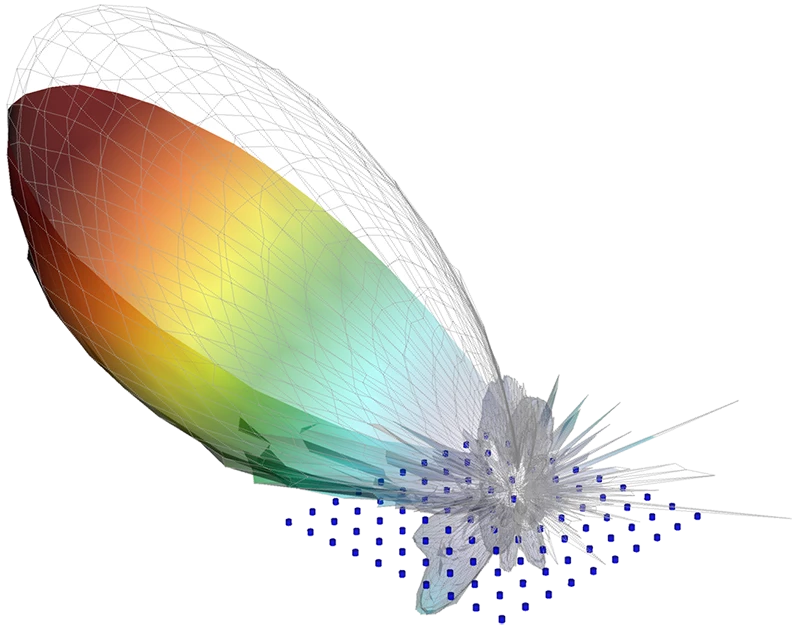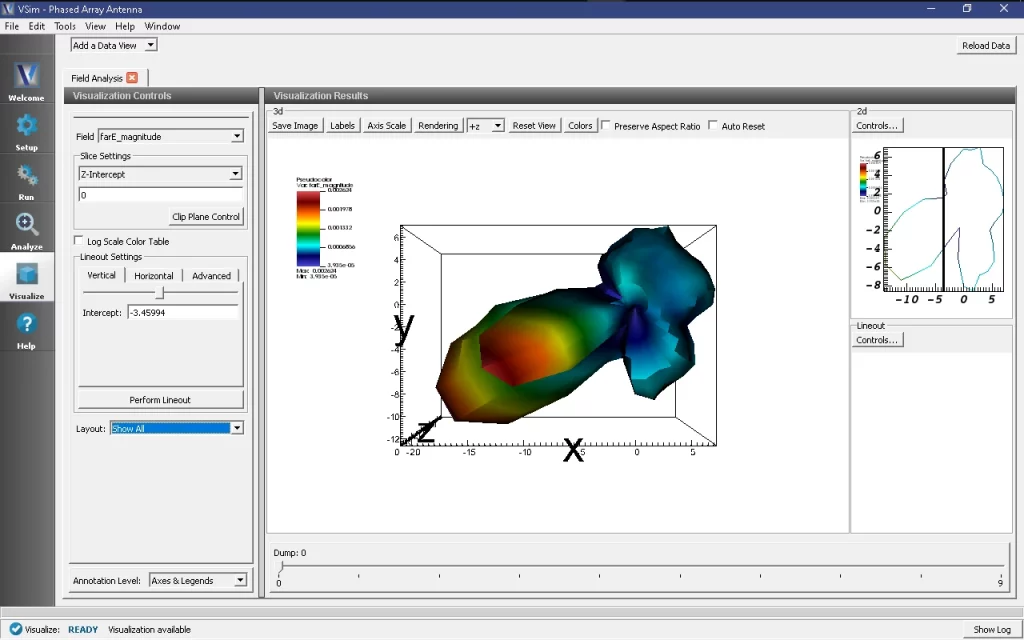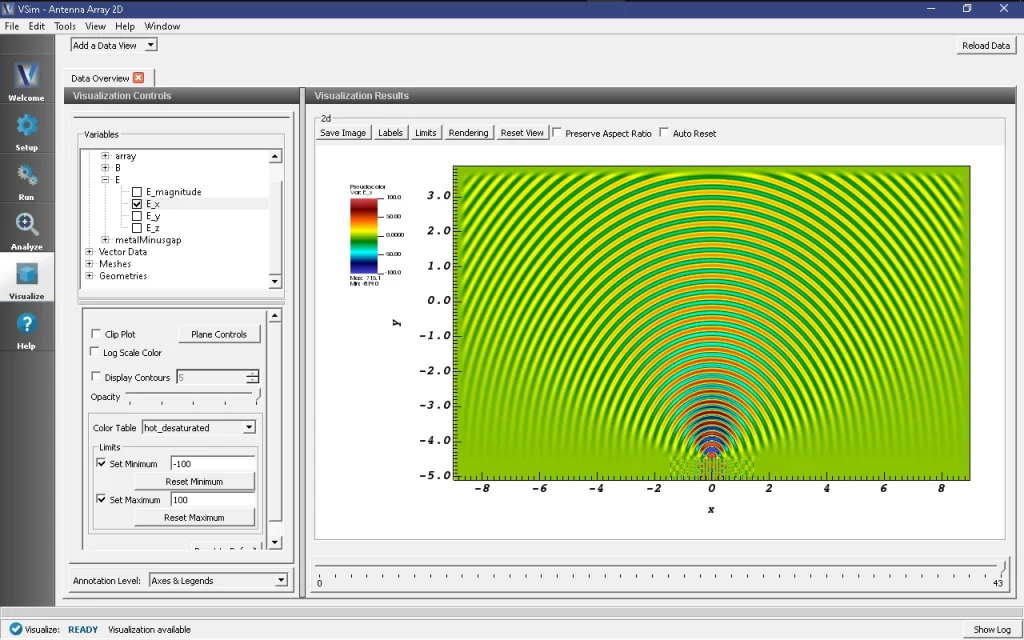# Phased Array Antennas

Phased array antennas are an essential component in the growth of 5G wireless systems because they provide more direct beamforming characteristics and steering than traditional single antennas. Engineers working to improve phased array antenna systems need simulation software that provides timely and accurate results. As an FDTD simulation tool, VSim can simulate multiple frequencies simultaneously, and only VSim implements a unique algorithm specialized for efficient far-field calculations.

• Accurate and Accurate Results

• Multiple Frequencies Simultaneously

Specialized algorithm for efficient far-field calculations

• Use built in geometries & Import CAD files

analyze antenna features• Beamforming solutions under different conditions with dielectrics

Kinetic plasma solver

• Simulate antennas with different radiation patterns

Measure coupling effects between array elements and reflections

• Calculate S-parameters for each array element

Create beamforming graphs

## Phased Array Example With VSimEMUsers can set up phased array antenna systems by using built-in geometries or importing CAD files.  VSim can be used to analyze antenna features and obtain beamforming solutions under different conditions with dielectrics, and even plasma with Tech-X’s kinetic plasma solver.

Users can observe radiation pattern changes based on:

• Geometry configuration
• Relative displacement between elements
• Excitation amplitude between elements
• Excitation phase between elements
• Coupling based on the pattern of individual elements

VSim users can perfect their antenna designs by measuring coupling effects between array elements and reflections, as well as calculate S-parameters for each array element and create beamforming graphs. To optimize design configurations, users can analyze performance results based on key elements such as: HPBW & FNBW, directivity, side-lobe levels, and more.

With VSimEM, users can set up antennas with different radiation patterns, frequencies, or polarizations within the same simulation, making it ideal for modeling MIMO antennas.0:09

4:04
Now it's to the left. We'll do a few more gums you can see the direction rotate around. And so that's basically what the simulation is about. Just how to set up what would be a standard phased array, how to get all the elements to add up to make a plane wave in one direction or or a beam

4:46
if you would in one direction. As I recall during the simulation, the direction rotates around one time during the course of this

5:30
Well, that was a moment we'll get. I seem to have dropped the connection there in my bath. You are back. Welcome back, David. That was a little scary. It must have been more data than it could handle. It is a sizable simulation. Okay, so I trust you all saw enough of that, that you know what's going on. So that's the cool part, that results are always very nice to look at. But the real topic of this talk is how, how do we make a simulation that does that. The first thing is geometry construction. Those of you who've used the software in the past know that you can create geometry by hand using shape primitives. The only problem with a something like a phased array is there could be hundreds of pieces of geometry. And you don't want to create them all by hand one at a time, because this would take days. So we have a basic a feature in our geometry creation called Create array that allows you to take a primitive shape. And then to duplicate that shape with a regular spacing, I'm going to try to show you how that works, I've got a open simulation here, just a totally empty simulation, nothing's been done yet. And I'm just going to show you how that works with creating arrays. So you basically we could add a primitives such as a cylinder here, that's a little too fat for me that cylinder. So I'm going to change the radius a little skinnier, we're going to change the direction, B in the z direction, which would match our simulation. And so now I have one cylinder pointing in the z direction. Maybe I can even spin it around. So now at this point, we can click and select this cylinder, and right mouse click and there is a feature called Create array. And this brings up a dialog box and allows you to create an array. So suppose I want a four by five array, one in the z direction, maybe my spacing is about point three, since my radius is about point one. Then I can accept that, and it will populate a set of arrays just like that. And so that's much faster. So that's 20 cylinders, all in a nice regular pattern. And it took one second to do, it would have taken an hour to do by hand kind of fix all those radii and orientation and all that stuff. So when you're doing a phased array, you will almost certainly be doing geometry construction with the Create array feature, unless of course you're importing a CAD file

9:08
now in our example, this is taken from our example that we'll be doing, we actually have a 15 by 15 array of short cylinders similar to what I did. This is a picture of what it looks like here. These cylinders have a small gap between them and the lower plane of the simulation. And they're assigned PVC material perfect electrical conducting material. In other words, they're metal so they're just little metal basically monopole antennas. Now, they have to the boundary conditions in any simulation on all six sides of your your rectangular domain. In this simulation, there are five open boundaries And one P E C boundary. We're using an open boundary here so that it takes up no space. Since this is a fairly sizable simulation, open boundaries allow a wave to go out. If they're in there perfect, it goes out perfectly if the wave is going out normal to the boundary, it's not so perfect if it's going out at an angle, but it's still pretty good. And you'll see you can see from our previous results, that there is very little reflection on these boundaries. Then on the lower plane, we have a infinite ground plane, which we set as a P E C boundary. And so if you look inside our field boundary conditions for this problem, you'll see those five open boundaries, and then you'll see a P E, C lower. And basically, it's a kind of PVC. And these, since these are actually infinitely thin boundaries on the domain boundaries, they do not display. And so unlike the metallic elements, the ground plane is not visible in the geo in the geometry window, but it is there as a boundary condition.

11:36
We'll need a source to drive all these 15 by 15. Little elements, we do it with a single source. So in the the field dynamics block, under current distributions, we just have a single source that allows for an arbitrary current, J XJY. And Jay Z in this case, JX and JY are zero, and we only have a jay z. So this is a current that runs from the ground plane up to the radiating elements. And we just assign it a spacetime function called caress t f, which you can see there. Will now obviously, we can't have the current come up through this whole value. So later on, you'll see how we mask out the current so that it only runs just below an element. But we do that with a mask function rather than trying to create 225 different sources. And the source itself will all be in a function called cur STF, which is x, y, z, x, y and time dependent.

13:14
So the hardest part of this example is all of these functions. If we go in and we look at the simulation, you'll see that there are functions and also the space time function. Under the space time function is our occur STF, which we previously saw was used in the current source. But this function is itself built up from many, several several other functions with various different names here. And so we have basically eight different functions to drive this. And so this is really where the wizardry happens for this example.

14:09

19:17
Functions part three, we saw that sine omega t plus k dot r that was all built into this phi function here. This is kind of got a little it's a little hard to figure but basically, we're representing our propagation constant K our wave number with a phi and theta angles in sort of a spherical coordinate system. In this simulation as we already saw, the azimuthal angle Got a very easy time. Whereas the elevation angle, I remains fixed at high force or 45 degrees from vertical. And theta runs from just two pi t over t off. So when you get to tee off, you run from zero to two pi. So that's the one time around. Now, if you play with this simulation yourself, we've kind of built in these bi and beta functions here, so that you can do anything you want. So you could have a fixed data, and you could have five Gaussian soil and have a beam that rocks up and down, for example, or you can do anything you want with these functions. The bottom line though, is you have to produce omega t plus k dot x or k dot r, it's what I've been using elsewhere. And so here's your omega oversee. So here's your K. Here's your if you represent this as a vector with these components, and here's your sine times your x cosine plus y sine, and we're at z equal to zero. And so we never see a cosine phi here in this function. And so this looks nasty, but it's really just omega t plus k dot out. Functions, part four in our amplitude we're using is a little bit fancy, we had that amplitude function in front. This is what it looks like in text form, it looks really scary. In the end, it's not that scary. You can see, whereas we had a x cosine theta y sine theta, we have an x sine theta minus y cosine theta. So that tells you we're kind of doing a cross product here. And so the actual formula we're using is a Gaussian. So it's basically distance divided by a with a sigma squared. But the two distances where we really have a product to two Gaussians. Here, the first Gaussian is a 1d Gaussian, perpendicular, or 2k. And so at low elevations, if we're kind of going out at quite an angle, this term will dominate. And what we get is a Gaussian in the width direction, but we use the full length of the array, so we don't put ours in the direction it's propagating. On the other hand, if we're doing a vertical direction going straight up, then we kind of just put an x squared plus y squared kind of width on it. And so we have sort of limited in two directions. And so yeah, this amplitude is a bit fancy. It's pretty typical though, where you want a one day Gaussian if you've got a low elevation B, and a 2d Gaussian if you've got a beam coming straight out of the array. Okay, so that's the source. And that's pretty much how this thing is set up. As you can see, most of the work went into the went into that function there. There is an extra element in this simulation, we have a history, which is a far field box. So if we go in here you're in our let's collapse that we have one history. And if you were to do it is a array history, our field box data. And this basically has a just XYZ, our arguments for this, and then an end tie a start time and an end time. And this

24:17
is the typical diagnostic we use whenever we want an array pattern. So whenever you see one of these DB versus angle kind of plots, in order to create that we have this history. Now the way this works is this just saves data on a on a box, the box has to surround the radiating elements. Technically, we should not have an infinite ground plane. But that still works fairly well with it there the low angles might be slightly off Since our elements are not very high above the plane, though, we'll get good results almost everywhere except right at very flattest angles. This history produces a lot of data. It is it's not something you can look at directly. However, it's designed to be used in conjunction with the analyzer Tam, and one of the analyzers called compute firefield from here called Fox. And here I've included a little blurb on the cure chaff theorem. This basically allows you to look at the fields on a surface that surrounds an antenna. And then to extrapolate those fields out to infinity. Or actually, we can this way, we have a ton, we can extrapolate to a finite radius. And that's what gives you your radiation pattern that you're so familiar with.

26:07
The way this looks when you run it is you go over to Analyze tab here, and you pick amongst all of your analyzers, and here it is compute our field from box, and up comes a little form to be filled out. The important things on the form are the theta and phi. And so these are the number of points on the fire sphere. And then you also pick the number of times on the fire sphere. And this is done as a time step strike a multipolar simulation time step, since you might have 100 time steps per period, you that's more data than you need. And so you might actually want only 20 time steps per period out on your fire sphere. And so then your time step strike would be five.

27:09
The result of this is you get radiation patterns. And so these are the instantaneous farfield patterns. Let's go to visualize. And once you do that, you may have to reload data. And then you'll see a new field called far e out here. And if we look at our E magnitude I think I probably have to take those off. Oh, that's because I've got contours on. It's always fun to do this. So here is a radiation. So I don't know if we can show on here the

28:19
see it's probably inside a pattern because our radius is 30 meters here. So you can see this is the pattern. And it's going up at about 45 degree angle. Off to the left there, you can see we have what would be called a grating lobe here a secondary lobe, which is undesirable, but often occurs. And sometimes optimization is about getting rid of that. We know grading loads go away if you use to bring your elements closer together, or if you use a lower frequency. And so as we run this simulation, these are instantaneous times. Or sometimes you're looking at a field pattern where the field is probably no out there. So you'll just see what's probably a bunch of garbage. And then along comes the next peak in the period. And then you have a strong peak which has been rotated because we're rotating and again we see our grading lobe here. And we can progress this through time. And see that each period we get a similar kind of pattern only continues to rotate around And so I think what I'll do is run one more time looking at the fields themselves. And that was the easy component. We were displaying contours. And we're running from minus seven to roughly minus eight plus eight volts per meter here, it's the magnitude is just whatever was was set. And usually the peaks are about accurate 10 higher than real field. So if you take a closer look, that's really what we've got.

30:47
I think I'll jump ahead a little bit. And I don't want the whole thing to go away. Again, it's a little slow here. But that's basically what this example looks like. So where would we use this? Well, like I I've said,

31:38